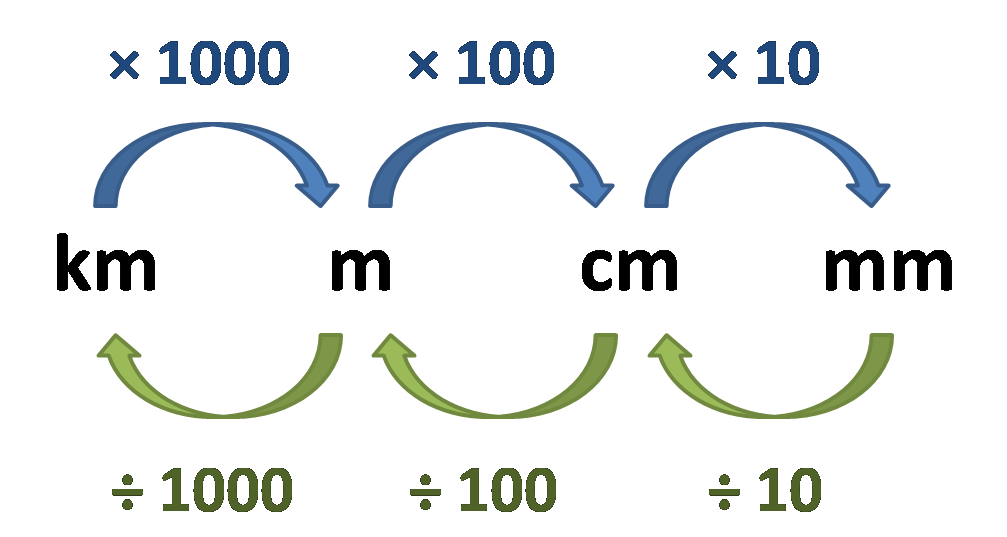# mm Calculator

Created by Arturo Barrantes
Reviewed by Julia Żuławińska
Last updated: Oct 27, 2023

The handy mm calculator helps you convert millimeters values to the other length units in the metric and imperial system. Here, we will cover what is a mm (millimetre) and how to convert mm to m, among other length units.

## What is a mm (millimetre)?

What does mm mean? The two letters mm stands for millimeter, and it's a unit for expressing length.

Remember everybody express their height in centimeters or in a combination of feet and inches, depending on where you live. Those values refer to the length of something, exactly as the millimetre.

How much is a millimeter? The mm is 1000th of a meter (m), and a meter is equal to 1/299,792,458 of the distance that light travels in a vacuum space in one second.

## How to convert mm to m?

Now that we know what mm means, we can use the following picture to describe how to convert millimeter to meters.You have to divide by ten and then by 100. Be careful with these factors; otherwise, you would end up converting millimeters to kilometers. In any case, you can check the result in our mm calculator.

Let's put an example: I want to know how many meters are in 1430 millimeters:

$\footnotesize \rm {1430 \ mm / 10 = 143 \ cm}$, then
$\footnotesize \rm {143 \ cm / 100 = 1.43 \ meters}$

Note how we followed the steps according to the picture above. You could do the same if you want to convert to cm, m, or km.

## How to convert mm to inches?

In this case, you have to remember that 1 inch equals 25.4 mm. Then if you have mm, you only have to divide it by 25.4. Imagine I want to express 1785 millimeters in inches; then,

$\footnotesize \rm {1785 \ mm / 25.4 = 70.28 \ inches}$

Our mm calculator offers a wide variety of possibilities, as you might have seen already: You can change mm to m, cm, km, in, ft, yd, mi, among others.

Besides of our mm calculator, here we want to mention other distance related calculators you could find useful:

## FAQ

### What is 1000th of a meter?

The 1000th of a meter equals 0.001 m, an inconvenient value because of the decimal part. Thus, we call express it in millimeters. The 1000th of a meter equals 1 mm.

### What is 3/8 inches to mm?

9.525 mm. Here's how we arrived at that answer:

1. Divide the numerator by the denominator. That is 0.375.
2. Multiply the results by the the conversion factor for inches to mm: 25.4.
3. Enjoy your distance measurement in the imperial weight system.
Arturo Barrantes
Input
mm
Metric
cm
m
km
Imperial/US
in
ft
yd
mi
People also viewed…

Addiction calculator tells you how much shorter your life would be if you were addicted to alcohol, cigarettes, cocaine, methamphetamine, methadone, or heroin.

### Chilled drink

With the chilled drink calculator, you can quickly check how long you need to keep your drink in the fridge or another cold place to have it at its optimal temperature. You can follow how the temperature changes with time with our interactive graph.

### Land area

Determine the area of any rectangular piece of land using this land area calculator.

### Quantity Converter

The quantity converter quickly alternates between nine quantity units, including dozen, score, and gross.